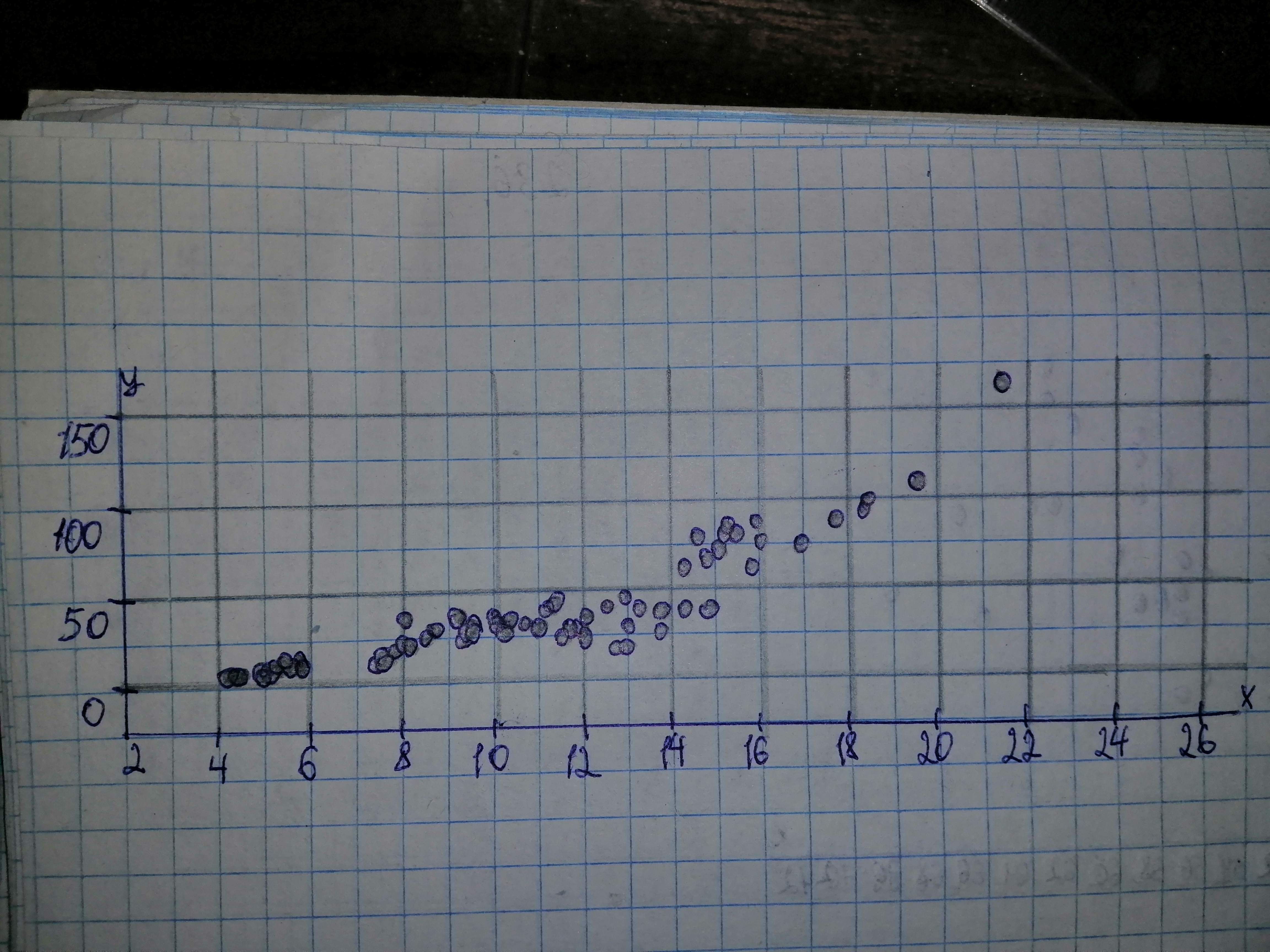Question# The ability to estimate the volume of a tree based on a simple measurement, such as the tree’s diameter, is important to the lumber industry, ecologis

Scatterplots
ANSWEREDThe ability to estimate the volume of a tree based on a simple measurement, such as the tree’s diameter, is important to the lumber industry, ecologists, and conservationists. Data on volume, in cubic feet, and diameter at breast height, in inches, for 70 shortleaf pines were reported in C. Bruce and F. X. Schumacher’s Forest Mensuration (New York: McGraw-Hill, 1935) and analyzed by A. C. Akinson in the article “Transforming Both Sides of a Tree” (The American Statistician, Vol. 48, pp. 307–312). a) Obtain a scatterplot for the data. b) Decide whether finding a regression line for the data is reasonable. If so, then also do parts (c)-(f). c) Determine and interpret the regression equation for the data. d) Identify potential outliers and influential observations. e) In case a potential outlier is present, remove it and discuss the effect. f) In case a potential influential observation is present, remove it and discuss the effect.2020-11-13

Given: $$\displaystyle{n}=\ \text{Sample size}\ ={70}$$ a) Diameter is on the horizontal axis and Volume is on the vertical axis.b) It is reasonable to find a regression lien for the data if there is no strong curvature present in the scatterplot. We note that there is no strong curvature in the scatterplot of part (a) and thus it is reasonable to find a regression line for the data. c) Let us first determine the necessary sums: $$\displaystyle\sum\ {x}_{{{i}}}={782.9}$$
$$\displaystyle\sum\ {{x}_{{{i}}}^{{{2}}}}={9934.65}$$
$$\displaystyle\sum\ {y}_{{{i}}}={2442.7}$$
$$\displaystyle\sum\ {x}_{{{i}}}{y}_{{{i}}}={35376.74}$$ Next, we can determine $$\displaystyle{S}_{{xx}}\ \text{and}\ {S}_{{{x}{y}}}$$
$$\displaystyle{S}_{{xx}}=\ \sum\ {{x}_{{{i}}}^{{{2}}}}\ -\ {\frac{{{\left(\sum\ {x}_{{{i}}}\right)}^{{{2}}}}}{{{n}}}}={9934.65}\ -\ {\frac{{{782.9}^{{{2}}}}}{{{70}}}}={1178.4727}$$
$$\displaystyle{S}_{{{x}{y}}}=\ \sum\ {x}_{{{i}}}{y}_{{{i}}}\ -\ {\frac{{{\left(\sum\ {x}_{{{i}}}\right)}{\left(\sum\ {y}_{{{i}}}\right)}}}{{{n}}}}={35376.74}\ -\ {\frac{{{782.9}\ \cdot\ {2442.7}}}{{{70}}}}={8056.8853}$$ The estimate b of the slope $$\displaystyle\beta\ \text{is the ratio of}\ {S}_{{{x}{y}}}\ \text{and}\ {S}_{{xx}}$$:
$$\displaystyle{b}=\ {\frac{{{S}_{{{x}{y}}}}}{{{S}_{{xx}}}}}=\ {\frac{{{8056.8853}}}{{{1178.4727}}}}={6.8367}$$ The mean is the sum of all values divided by the number of values: $$\displaystyle\overline{{{x}}}=\ {\frac{{\sum\ {x}_{{{i}}}}}{{{n}}}}=\ {\frac{{{782.9}}}{{{70}}}}={11.1843}$$
$$\displaystyle\overline{{{y}}}=\ {\frac{{\sum\ {y}_{{{i}}}}}{{{n}}}}=\ {\frac{{{2442.7}}}{{{70}}}}={34.8957}$$ The estimate a of the intercept $$\displaystyle\alpha$$ is the average of y decreased by the product of the estimate of the slope and the average of x. $$\displaystyle{a}=\ \overline{{{y}}}\ -\ {b}\ \overline{{{x}}}={34},{8957}\ -\ {6},{8367}\ \cdot\ {11},{1843}=\ -{41},{5681}$$ General least-squares equation: $$\displaystyle\hat{{{y}}}=\ \alpha\ +\ \beta\ {x}\ \text{Replace}\ \alpha\ \text{by}\ {a}=\ -{41.5681}\ \text{and}\ \beta\ \text{by}\ {b}={6.8367}$$ in the general least-squares equation: $$\displaystyle{y}={a}\ +\ {b}{x}=\ -{41.5681}\ +\ {6.8367}{x}$$ d) There appear to be one outliers, because the point in the top right corner of the scatterplot list more to the right than all other points in the scatterplot. There appear to be no influential observations beside the outlier, because all data values lie near the regression line except fro the outlier. e) Let us first determine the necessary sums: $$\displaystyle\sum\ {x}_{{{i}}}={759.5}$$
$$\displaystyle\sum\ {{x}_{{{i}}}^{{{2}}}}={9387.09}$$
$$\displaystyle\sum\ {y}_{{{i}}}={2279.2}$$
$$\displaystyle\sum\ {x}_{{{i}}}{y}_{{{i}}}={31550.84}$$ Next, we can determine $$\displaystyle{S}_{{xx}}\ \text{and}\ {S}_{{{x}{y}}}$$
$$\displaystyle{S}_{{xx}}=\ \sum\ {{x}_{{{i}}}^{{{2}}}}\ -\ {\frac{{{\left(\sum\ {x}_{{{i}}}\right)}^{{{2}}}}}{{{n}}}}={9387.09}\ -\ {\frac{{{759.5}^{{{2}}}}}{{{69}}}}={1027.0864}$$
$$\displaystyle{S}_{{{x}{y}}}=\ \sum\ {x}_{{{i}}}{y}_{{{i}}}\ -\ {\frac{{{\left(\sum\ {x}_{{{i}}}\right)}{\left(\sum\ {y}_{{{i}}}\right)}}}{{{n}}}}={31550.84}\ -\ {\frac{{{759.5}\ \cdot\ {2279.2}}}{{{69}}}}={6463.1241}$$ The estimate b of the slope $$\displaystyle\beta\ \text{is the ratio of}\ {S}_{{{x}{y}}}\ \text{and}\ {S}_{{xx}}$$:
$$\displaystyle{b}=\ {\frac{{{S}_{{{x}{y}}}}}{{{S}_{{xx}}}}}=\ {\frac{{{6463.1241}}}{{{1027.0864}}}}={6.2927}$$ The mean is the sum of all values divided by the number of values: $$\displaystyle\overline{{{x}}}=\ {\frac{{\sum\ {x}_{{{i}}}}}{{{n}}}}=\ {\frac{{{759.5}}}{{{69}}}}={11.0072}$$
$$\displaystyle\overline{{{y}}}=\ {\frac{{\sum\ {y}_{{{i}}}}}{{{n}}}}=\ {\frac{{{2279.2}}}{{{69}}}}={33.0319}$$ The estimate a of the intercept $$\displaystyle\alpha$$ is the average of y decreased by the product of the estimate of the slope and the average of x. $$\displaystyle{a}=\ \overline{{{y}}}\ -\ {b}\ \overline{{{x}}}={33.0319}\ -\ {6.2927}\ \cdot\ {11.0072}=\ -{36.2332}$$ General least-squares equation: $$\displaystyle\hat{{{y}}}=\ \alpha\ +\ \beta\ {x}\ \text{Replace}\ \alpha\ \text{by}\ {a}=\ -{36.2332}\ \text{and}\ \beta\ \text{by}\ {b}={6.2927}$$ in the general least-squares equation: $$\displaystyle{y}={a}\ +\ {b}{x}=\ -{36.2332}\ +\ {6.2927}{x}$$ We note that the regression line is slightly less steep than the regression line in part (a) and thus the outlier makes the regression slightly steeper. f) Let us first determine the necessary sums: $$\displaystyle\sum\ {x}_{{{i}}}={759.5}$$
$$\displaystyle\sum\ {{x}_{{{i}}}^{{{2}}}}={9387.09}$$
$$\displaystyle\sum\ {y}_{{{i}}}={2279.2}$$
$$\displaystyle\sum\ {x}_{{{i}}}{y}_{{{i}}}={31550.84}$$ Next, we can determine $$\displaystyle{S}_{{xx}}\ \text{and}\ {S}_{{{x}{y}}}$$
$$\displaystyle{S}_{{xx}}=\ \sum\ {{x}_{{{i}}}^{{{2}}}}\ -\ {\frac{{{\left(\sum\ {x}_{{{i}}}\right)}^{{{2}}}}}{{{n}}}}={9387.09}\ -\ {\frac{{{759.5}^{{{2}}}}}{{{69}}}}={1027.0864}$$
$$\displaystyle{S}_{{{x}{y}}}=\ \sum\ {x}_{{{i}}}{y}_{{{i}}}\ -\ {\frac{{{\left(\sum\ {x}_{{{i}}}\right)}{\left(\sum\ {y}_{{{i}}}\right)}}}{{{n}}}}={31550.84}\ -\ {\frac{{{759.5}\ \cdot\ {2279.2}}}{{{69}}}}={6463.1241}$$ The estimate b of the slope $$\displaystyle\beta\ \text{is the ratio of}\ {S}_{{{x}{y}}}\ \text{and}\ {S}_{{xx}}$$:
$$\displaystyle{b}=\ {\frac{{{S}_{{{x}{y}}}}}{{{S}_{{xx}}}}}=\ {\frac{{{6463.1241}}}{{{1027.0864}}}}={6.2927}$$ The mean is the sum of all values divided by the number of values: $$\displaystyle\overline{{{x}}}=\ {\frac{{\sum\ {x}_{{{i}}}}}{{{n}}}}=\ {\frac{{{759.5}}}{{{69}}}}={11.0072}$$
$$\displaystyle\overline{{{y}}}=\ {\frac{{\sum\ {y}_{{{i}}}}}{{{n}}}}=\ {\frac{{{2279.2}}}{{{69}}}}={33.0319}$$ The estimate a of the intercept $$\displaystyle\alpha$$ is the average of y decreased by the product of the estimate of the slope and the average of x. $$\displaystyle{a}=\ \overline{{{y}}}\ -\ {b}\ \overline{{{x}}}={33.0319}\ -\ {6.2927}\ \cdot\ {11.0072}=\ -{36.2332}$$ General least-squares equation: $$\displaystyle\hat{{{y}}}=\ \alpha\ +\ \beta\ {x}\ \text{Replace}\ \alpha\ \text{by}\ {a}=\ -{36.2332}\ \text{and}\ \beta\ \text{by}\ {b}={6.2927}$$ in the general least-squares equation: $$\displaystyle{y}={a}\ +\ {b}{x}=\ -{36.2332}\ +\ {6.2927}{x}$$ We note that the regression line is slightly less steep than the regression line in part (a) and thus the potential influential observation makes the regression slightly steeper.